# Using Regression Kriging to Analyze Groundwater According to Depth and Capacity of Wells

1Department of Statistic and Informatics, College of Administration and Economics, Sulaimani University, Sulaimani City, Kurdistan Region – Iraq; 2Department of Accounting, College of Administration and Economics, Human Development University, Sulaimani City, Kurdistan Region – Iraq

Corresponding author’s e-mail: Aras Jalal Mhamad, Department of Statistic and Informatics, College of Administration and Economics, Sulaimani University, Sulaimani City, Kurdistan Region – Iraq, Department of Accounting, College of Administration and Economics, Human Development University, Sulaimani City, Kurdistan Region – Iraq. E-mail: aras.mhamad@univsul.edu.iq
Received: 20-04-2019 Accepted: 22-05-2019 Published: 29-05-2019
DOI: 10.21928/uhdjst.v3n1y2019.pp39-47

ABSTRACT

Groundwater is valuable because it is needed as fresh water for agricultural, domestic, ecological, and industrial purposes. However, due to population growth and economic development, the groundwater environment is becoming more and more important and extensive. The study contributes to current knowledge on the groundwater wells prediction by statistical analysis under-researched. Such as, it seems that the preponderance of empirical research does not use map prediction with groundwater wells in the relevant literature, especially in our region. Instead, such studies focus on several simple statistical analysis such as statistical modeling package. Accordingly, the researcher tried to use the modern mechanism such as regression kriging (RK), which is predicted the groundwater wells through maps of Sulaimani Governorate. Hence, the objective of the study is to analyze and predicting groundwater for the year 2018 based on the depth and capacity of wells using the modern style of analyzing and predicting, which is RK method. RK is a geostatistical approach that exploits both the spatial variation in the sampled variable itself and environmental information collected from covariate maps for the target predictor. It is possible to predict groundwater quality maps for areas at Sulaimani Governorate in Kurdistan Regions Iraq. Sample data concerning the depth and capacity of groundwater wells were collected on Groundwater Directorate in Sulaimani City. The most important result of the study in the RK was the depth and capacity prediction map. The samples from the high depth of wells are located in the South of Sulaimani Governorate, while the north and middle areas of Sulaimani Governorate have got low depths of wells. Although the samples from the high capacity are located in the South of Sulaimani Governorate, in the north and middle the capacity of wells have decreased. The classes (230–482 m) of depth are the more area, while the classes (29–158 G/s) of capacity are the almost area in the study.

Index Terms: Groundwater Analysis, Interpolation, Regression Kriging

### 1. INTRODUCTION

Groundwater is a valuable freshwater resource and constitutes about two-third of the fresh water reserves of the world . Buchanan (1983) estimated that the groundwater volume is 2000 times higher than the volume of waters in all the world’s rivers and 30 times more than the volume contained in all the fresh water of the world lakes. The almost is 5.0 L × 1024 L in the world of groundwater reservoir . Groundwater is used in many fields for industrial, domestic, and agricultural purposes. However, due to the population growth and economic development, the groundwater environment is becoming more and more important and extensive , and the heavy groundwater extraction has caused many problems such as groundwater level drop, saltwater intrusion, and ground surface depression, which need to be improved. Therefore, the identification, assessment, and remediation of groundwater problems have become quite a crucial and useful topic in the current time. For the above reasons, the analysis of groundwater requires implementing scientific and academic methods, from which one of the verified models is the RK that is used for this purpose . Regression-kriging (RK) is a spatial prediction technique that combines regression of the dependent variable on auxiliary variables with kriging of the regression residuals. It is mathematically a consideration of interpolation method variously called universal kriging and kriging with external drift, where auxiliary predictors are used to solve the kriging weights directly . RK is an application of the best linear unbiased predictor for spatial data, which is the best linear interpolator assuming the universal model of spatial variation . RK is used in many fields, such as soil mapping, geological mapping, climatology, meteorology, species distribution modeling, and some other similar fields . Regression kriging (RK) is one of the most widely used methods, which uses hybrid techniques and combines ordinary kriging with regression using ancillary information. Since the correlation between primary and secondary variables is significant , so, the aim of this study is to analyze and predicting groundwater depending on depth and capacity of wells in Sulaimani Governorate; using RK.

### 1.1. Objective of the Study

The main objective of this research is to analyze and predict groundwater wells at the un-sampled locations in Sulaimani Governorate according to depth and capacity of existing groundwater wells using RK and to assess the accuracy of these predictions.

### 2.1. Interpolation

Spatial interpolation deals with predicting values of the locations that have got unknown values. Measured values can be used to interpolate, or predict the values at locations which were not sampled. In general, there are two accepted approaches to spatial interpolation. The first method uses deterministic techniques in which only information from the observation point is used. Examples of direct interpolation techniques are such as inverse distance weighting or trend surface estimation. The other method depends on regression of addition information, or covariates, gathered about the target variable (such as regression analysis combined with kriging). These are geostatistical interpolation techniques, better suited to count for spatial variation, and capable of quantifying the interpolation errors. Hengl et al. (2007) advocate the combination of these two into so-called hybrid interpolation. This is known as RK . In another paper, Hengl et al. (2004) explain a structure for RK, which forms the basis for the research in this study . Limitation of RK is the greater complexity than other more straightforward techniques like ordinary kriging, which in some cases might lead to worse results .

### 2.2. RK

The most basic form of kriging is called ordinary kriging. When we add the relationship between the target and covariate variables at the sampled locations and apply this to predicting values using kriging at unsampled locations, we get RK. In this way, the spatial process is decomposed into a mean and residual process. Thus, the first step of RK analysis is to build a regression model using the explanatory grid maps . The kriging residuals are found using the residuals of the regression model as input for the kriging process. Adding up the mean and residual components finally results in the RK prediction . RK is a combination of the traditional multiple linear regression (MLR) and kriging, which means that an unvisited location s0 is estimated by summing the predicted drift and residuals. This procedure has been found preferable for solving the linear model coefficients  and has been applied in several studies. The residuals generated from MLR were kriged and then added to the predicted drift, obtaining the RK prediction. The models are expressed as:

When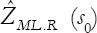is the predicted value of the target variable z at location s0 using MLR model,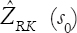is the predicted value of the target variable at location s0 using RK model, is the regression coefficiency for the kth explanatory variable Xk, p is the total number of explanatory variables, wi (s0) are weights determined by the covariance function and e (si) are the regression residuals. In a simple form, this can be written as:

When z(s) is the value of a phenomenon at location s, m(s) is the mean component at s, and ε′ (s) stands for the residual component including the spatial noise. The mean component is also known as the regression component.

The process of refining the prediction in two steps (trend estimation and kriging) is shown in Fig. 1, where the result of the mean component, only regression, is visible as a dashed line, and the sum of trend + kriging is the curving thick line . This should approach the actual distribution better than either just a trend surface or a simple interpolation. The linear modeling of the relationship between the dependent and explanatory variables is quite empirical. The model selection determines which covariable is important, and which one is not. It is not necessary to know all these relations, as long as there is a significant correlation. Once the covariates have been selected, their explanatory strength is determined using (stepwise) MLR analysis. For each covariate this, leads to a coefficient value, describing its predictive strength, and whether this is a positive or negative relationship. With the combination of values for all covariate maps, a trend surface is constructed. This regression prediction is, in fact, the calculation for each target cell from each input cell from all covariates times the coefficient value. The amount of correlation is expressed by R2 in the regression equation. To enable this, the covariate data first need to be processed by overlaying the sample locations with the covariate data layers. In this way, a matrix of covariate values for each sample point is constructed. This matrix may still hold several “NA” or missing values due to the fact that some maps do not have coverage, while some others do. An example of this is the absence of information on the organic matter in urban areas. Since the linear models cannot be constructed properly when some covariate data are missing, these sample points are discarded altogether. The resulting data matrix is therefore complete for all remaining measurement data points. The second step in which the covariate data are needed is the model prediction phase of the mean surface values. First, a prediction mask is made, which is the selection of grid cells for which covariate data are available and only contains the coordinates of valid cells. Next, the regression mean values are calculated by predicting the regression model for every grid cell in the prediction mask. In the residual kriging phase, this prediction grid is used again as a mask for the kriging prediction .

Fig. 1. A schematic representation of regression kriging using a cross-section .

### 2.3. Variogram and Semivariogram

Semivariogram analysis is used for the descriptive analysis. The spatial structure of the data is investigated using semivariogram. This structure is also used for predictive applications, in which the semivariogram is used to fit a theoretical model, parameterized, and also used to predict a regionalized variable at other unmeasured points. Estimating the mean function X(s)Tβ and the covariance structure of ε(s) for each s in the area of interest is the first step in both the analysis of the spatial variation and the prediction. Semivariogram is commonly used as a measure of spatial dependency. The estimated semivariogram explains a description of how the data is correlated with the distance. The factor 1/2 that ϒ(h) indicates is a semivariogram, and 2ϒ(h) is the variogram. Thus, the semivariogram function measures half the average squared difference between pairs of data values separated by a given distance, h, which is known as the lag , . The experimental variogram is a plot of the semivariance against the distance between sampling points. The variogram is the fitted line that best describes the function connecting the dots from the experimental variogram . Assuming that the process is stationary, the semivariogram is defined in equation (4):

Here, N(h) is the set of all pairwise Euclidean distances i–j = h, Nh is the number of distinct pairs in N(h). Z(si) and z(sj) are the values at spatial location i and j, respectively, and ϒ(h) is the estimated semivariogram value at distance h.

The semivariogram has three important parameters: The nugget, sill, and range. The nugget is a scale of sub-grid variation or measurement error, and it is indicated by the intercept graphically. The sill is the semivariant value as the lag (h) goes to infinity, and it is equal to the total variance of the data set. The range is a scalar which controls the degree of correlation between data points (i.e., the distance at which the semivariogram reaches its sill). As shown in Fig. 2, it is then necessary to select a type of theoretical semivariogram model based on that estimate. Commonly used theoretical semivariogram shapes increase monotonically as a function of distance, by comparing the plot of empirical semivariogram with various theoretical models that can choose the semivariogram model. Three are some parametric semivariogram models for testing, such as: Exponential, gaussian, and spherical. These models are given by the following equations:

Fig. 2. Illustration of semivariogram parameters.

Gaussian:

Spherical:

When h is a spatial lag, θ0 is the nugget, θ1 is the spatial variance (also referred to as the sill), and θ2 is the spatial range. The nugget, sill, and range parameters of the theoretical semivariogram model can fit the empirical semivariogram ϒ(h) by minimizing the nonlinear function. When fitting a semivariogram model, if we consider the empirical semivariogram values and try to fit a model to them as a function of the lag distance h, the ordinary least squares’ function is as given by,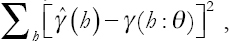where ϒ(h: θ) denotes the theoretical semivariogram model and θ = (θ0, θ1, θ2) is a vector of parameters. RK computes the parameters θ and β separately. The parameters β in the mean function are estimated by the least squares method. Then, it computes the residuals, and their parameters in the semivariogram are estimated by various estimation methods, such as least squares or a likelihood function. Prediction of RK at a new location s0 can be performed separately using a regression model to predict the mean function, a kriging model of prediction residuals and then adding them back together as in Equation (8):

Here, si = (xi, yi) is the known location of the ith sample, xi and yi are the coordinates, βk is the estimated regression model coefficient, λi represents the weight applied to the ith sample (determined by the variogram analysis), ε(si) represents the regression residuals, and X1(s0)… Xn(s0) are the values of the explanatory variables at a new location s0. The weight λi is chosen such that the prediction error variance is minimized, yielding weights that depend on the semivariogram . More details about the kriging weight λi follow immediately .

The main objective is to predict Z(s) at a location known as s0, given the observations {Z(s1), Z(s2),…, Z(s3)}′. For simplicity we assume E{Z(s)} = 0 for alls. We briefly outline the derivation of the widely used kriging predictor. Let the predictor be in the form of, where λ = {λ1, λ2,…, λn}′. The objective is to find weights λ, which is a minimum.

By minimizing Q(s0) with respect to λ, it can be shown that;

When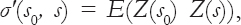, and ∑= E[Z(s) Z (s)] are the covariance matrix. The minimum of Q(s0) is min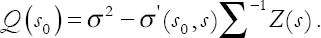. Note that, Q(s0) can be rewritten in terms of the variogram by applying;

When Γ(s0, s) is the corresponding matrix of variograms. We can thus rewrite Q(s0) given in Equation (9) as;

Q(s0) is now minimized with respect to λ, subject to the constraint λ′ 1 = 1 (accounting for the unbiasedness of the predictor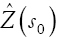) .

Geostatistical techniques such as multiple regression, inverse distance weight, simple kriging, and ordinary kriging uses either the concept of regression analysis with auxiliary variables or kriging for prediction of target variable, whereas RK is a mixed interpolation technique; it uses both the concepts of regression analysis with auxiliary variables and kriging (variogram analysis of the residuals) in the prediction of target variable. It considers both the situations, i.e., long-term variation (trend) as well as local variations. This property of RK makes it superior (more accurate prediction) over the above-mentioned techniques . Among the Hybrid interpolation techniques, RK has an advantage that there is no danger of instability as in the kriging with the external drift . Moreover, the RK procedure explicitly separates the estimated trend from the residuals and easily combined with the general additive modeling and regression trees [16,17].

### 2.5. Cross-Validation of RK Results

To assess which spatial prediction method provides the most accurate interpolation method, cross-validation is used to compare the estimated values with their true values. Cross-validation is accomplished by removing each data point and then using the remaining measurements to estimate the data value. This procedure is repeated for all observations in the dataset. The true values are subtracted from the estimated values. The residuals resulting from this procedure are then evaluated to assess the performance of the methods. One particular method is called k-fold cross-validation, where k stands for the number of folds one wants to apply. Each fold is a set of data kept apart from the analysis, repeated for the number of folds. A special type of k-fold cross validation is where the repetition of analyses (k) is equal to the number of data. This is called leave one out cross-validation, for the analysis is repeated once for every sample in the dataset, omitting the sample value itself. Resulting is a prediction for every observation, made using the same variogram model settings as for the normal RK prediction. The degree in which the cross-validation predictions resemble the observations is then a measure for the goodness of the prediction method. This can be calculated using the mean squared normalized error or “z score” . To aid further in the assessment of prediction results, additional parameters can be calculated from the cross-validation output, such as the mean prediction error (MPE), root mean square prediction error (RMSPE), and average kriging standard error (AKSE).

When N stands for the number of pairs of observed and predicted values, Z(x) is the observed value at location x, and z’(x) is the predicted value by ordinary kriging at location z.

Here, x is a location, and σ(x) is the prediction standard error for location x. MPE indicates whether a prediction is biased and should be close to zero. RMSPE and AKSE are measures of precision and have to be more or less equal. The cv-procedure only accounts for the kriging part, since the input is the residuals from the linear modeling phase .

### 3.1. Data Description

Data were obtained from a (groundwater directorate/well license department) in Sulaimani, Kurdistan-Region. 451 observations (wells) were used in the study, only records containing valid x, y – locations are used in the statistical modeling process. One check is to print all measurement locations to check whether they are located within the defined regions. If not, they are removed. The RK method is suitable for predicting the groundwater wells, due to nature of data (there are coordinates for each wells). For kriging purposes, duplicate x,y-locations need to be checked, to prevent singularity issues, as shown by Yang et al. . Duplicated locations share the same coordinates (based on one decimal digit), making it impossible to apply interpolation. Therefore, the choice is made to delete each second record that has duplicated coordinates. The research area is limited to the Sulaimani Governorate of the Kurdistan region, only depth and capacity of wells are available at the individual point locations. Therefore, this research is targeted at depth and capacity of wells. The data were presented in Fig. 3. The dataset used for the analysis contains six variables and deals with properties of well for the year 2018, which are (depth, capacity, state well level, dynamic well level, latitude-X, and longitude-Y).

Fig. 3. Sample distribution.

### 3.2. Experimental Variogram

Once the regression prediction has been performed, the variogram for the resulting residuals from the sample data can be modeled, as shown in Fig. 4.

Fig. 4. Variogram for the depth of the wells.

The model of depth with partial sill C0 = 5328, nugget = 371.95, and range = 0.078 was used for the residual variogram. The result has indicated that with an increase in distance, the semivariance value increases. Semivariograms are used to fit the residuals of the recharge estimates to enable the residuals then to be spatially interpolated by kriging. Fig. 4 shows simple kriging of the modeled residuals using the same locations from the first prediction surface; the kriging is provided over the surface to obtain the results, which are not interpolated over geological boundaries, which are not necessary to have any spatial correlation with the residuals. These semivariograms explain the nugget that is high in each group. When the nugget value is high, it indicates low spatial correlation in the residuals and has an effect that interpolation is not trying to match each point value of the residuals. Although the range shows the extent of the spatial correlation of recharge residuals, it ensures that the residuals’ spatial surface is only using the local information.

The model for capacity of wells has partial sill C0 = 3805.4, nugget = 11222.3, and range = 0.429. The result has indicated that with an increase in distance, the semivariance value increases. Fig. 5 shows simple kriging of the modeled residuals using the same locations from the first prediction surface for the capacity of wells. From the semivariograms, the nugget also is high in each group, which indicates low spatial correlation in the residuals. The range shows the extent of the spatial correlation of recharge residuals and ensures that the residuals’ spatial surface is only using the local information.

Fig. 5. Variogram for the capacity of wells.

From Fig. 6, the variance has some artifacts. It can be expected that values close to the locations, where point samples were taken, have lower variances. However, the blue colored regions in the depth of wells appear very strange here, especially when other sparsely sampled regions do not have this blue color, but yellow and orange colors, indicating a lower variance value. The kriging variance is produced together with the kriging operation and is shown in the left part of Fig. 6. Although in the prediction map, the blue areas correspond somewhat to higher predictions for depth wells (red, 432–482 m Fig. 6 - left), this is not reversely so for the other regions. There are some points located at the blue area, each having a high depth of wells (ranged between 432 and 482 m). In the blue area, this phenomenon is enlarged, showing the scale at which the variance is increasing in the depth of wells, just around a cluster of sample points at the blue area. It is observed from the predicted depth of wells that the values are higher in the Kalar, Kifri, and Khanaqin (lower portion of the study area), followed by Sulaimani Governorate, while low values are found in the upper of Sulaimani Governorate (upper portion of the study area). This fact can be seen from the RK variance (Fig. 6 - left). Higher variance values (482) for the depth of wells are found in the plain areas whereas the mountainous areas have relatively lower values (29.7).

Fig. 6. Regression kriging results (left) and variance (right) of the residuals from the depth of wells’ model.

Fig. 7 explains the variance. It can be expected that values have lower variances, close to the locations, where point samples were taken. Although the blue colored regions in the capacity of wells’ appear have very high variance value, yellow and orange colors are indicating a lower variance value. The kriging variance is produced together with the kriging operation and is shown in the left part of Fig. 7. Although in the prediction map, the blue areas correspond with higher predictions for capacity wells (372–415 G/m Fig. 7 - right). There are some points located at the blue area, each having high capacity of wells (range 372–415 G/m). In the blue area, this phenomenon is enlarged, showing the scale at which the variance is increasing in the capacity of wells. It is observed from the predicted capacity of wells that the values are higher in Kalar, Kifri, and Khanaqin, followed by Sulaimani Governorate, while low values are found in the upper of Sulaimani Governorate. This fact can be seen from the RK variance (Fig. 7 - left). Higher variance values (415) are found in the plain areas, whereas the mountainous areas have relatively lower values (29.8).

Fig. 7. Regression kriging results (left) and variance (right) of the residuals from the capacity of wells’ model.

### 3.3. Cross-validation of Kriging

Cross-validation was used to obtain the goodness of fit for the model. In addition, for each cross-validation result, the MPE, or mean prediction error, was calculated. The MPE-value should be close to zero. RMSPE (root mean square prediction error) and AKSE are given as well. The latter two error values should be close to each other, indicating prediction stability. The validation points were collected from all data in the study area so as to have an unbiased estimated accuracy. In this study, MPE, RMSPE, and AKSE are the three statistical parameters used for validation. The smaller the RMSPE, means the closer the predicted values to the observed values. Similarly, the MPE gives the mean of residuals and the unbiased prediction gives a value of zero. The results of the validation analysis are summarized in Table 1.

TABLE 1 Cross-validation results

The MPE is quite low in both depth and capacity of wells and is a low bias value of 0.019 and 0.021, respectively. The value of MPE is a result of a slight over-estimation of predicted depth and capacity of wells in the model. The RMSPE value is only 0.844 and 1.31, indicating the closeness of predicted value with the observed value. The results indicate the utility of RK in spatially predicting depth and capacity of wells even in the varying landscape.

### 4. RESULTS AND CONCLUSIONS

The results of the study show that the cross-validation measurement of the models was achieved. Looking at the quantitative results from the cross-validation, there are no obvious indications that the kriging model prediction is worse in the models of depth and capacity of wells. One important result of the study is the region model predictions in the dataset with sample values. The samples from the high depth of wells are almost absent in north and middle of Sulaimani Governorate, while in the south they are present, although the capacity of wells gave the same result depth of the wells. The samples from the high capacity of wells are almost absent in the north and middle of Sulaimani Governorate, while in the south they are present. In the map results after the kriging in Figs. 4 and 5, the areas within class (230–482m) of depth are almost, this result was close to the master thesis from Iraq – Sulaimani University by Renas Abubaker Ahmed, 2014, in which resulted that the depth of wells was between 20 m and more the 170 m for the same areas, which was used multivariate adaptive regression spline model to predicting groundwater wells , while the areas within class (29–158 G/s) of capacity are almost in the study, also it was close to Miss. Rena’s results, which is reported that the capacity of wells between 10 and 140 gallon .

### REFERENCES

. D. Wu and P. Lorenz. “The internet of things for smart cities:Technologies and applications”. IEEE Network, vol. 33, 4-5, 2019.

. I. Lee and K. Lee. “The internet of things (IoT):Applications, investments, and challenges for enterprises”. Business Horizons, vol. 58, no. 4, pp. 431-440, 2015.

. F. Wang and T. Su. “Indoor tracking by rfid fusion with IMU data”. Asian Journal of Control, vol. 21, no. 2, pp. 1-10, 2019.

. A. A. R. Green. “RFID Pet Monitoring and Identification System with RFID Tag Access Hatch”. Universiry of West London, London, 21389570, 2019.

. R. Tesoriero, J. A. Gallud, M. D. Lozano and V. M. R. Penichet. “Tracking autonomous entities using RFID technology”. IEEE Transactions on Consumer Electronics,vol. 55, no. 2, pp. 650-655, 2009.

. “Types of Rfid Systems”, 2018. Available from:https://www.impinj.com/about-rfid/types-of-rfid-systems. [Last accessed on 2019 Feb 20].

. Dejan. ”How To Build an Arduino Wireless Network with Multiple NRF24L01 Modules How to mechatronics”, 2019. Available from:https://www.howtomechatronics.com/tutorials/arduino/how-to-build-an-arduino-wireless-network-with-multiple-nrf24l01-modules. [Last accessed on 2019 Mar 10].

. R. Agrawal. Integrated Parallel K-Nearest Neighbor Algorithm. Springer, Singapore.

. M. A. Jabbar, B. L. Deekshatulu, and P. Chandra. “Classification of Heart Disease Using K Nearest Neighbor and Genetic Algorithm”. Procedia Technology, vol. 10, 85-94, 2013.

. S. Buczkowska, N. Coulombel and M. De Lapparent. A comparison of Euclidean Distance, Travel Times, and Network Distances in Location Choice Mixture Models. Networks and Spatial Economics, Berlin, 2019.

. R. Kohavi. “A Study of Cross-Validation and Bootstrap for Accuracy Estimation and Model Selection”. Proceeding IJCAI'95 Proceedings of the 14th International Joint Conference on Artificial Intelligence. vol. 2, 1137-1143, 2013.

. M. H. Asghar, A. Negi and N. Mohammadzadeh. “Principle application and vision in Internet of Things (IoT)”. International Conference on Computing, Communication and Automation, pp. 427-431, 2015.

. A. Sharma and R. Kumar. “Service-level agreement energy cooperative quickest ambulance routing for critical healthcare services”. Arabian Journal for Science and Engineering, vol. 44, no. 4, pp. 3831-3848, 2019.

. R. Pi and K. Zeb. “Healthcare Monitoring System and Transforming Monitored Data Into Real Time Clinical Feedback Based on Iot Using”. 2019 2nd International Conference on Computing, Mathematics and Engineering Technologies (iCoMET),pp. 1-6, 2019.

. A. Subasi, M. Radhwan, R. Kurdi and K. Khateeb. “IoT Based Mobile Healthcare System for Human Activity Recognition”. 2018 15th Learning and Technology Conference,pp. 29-34, 2018.

. B. Y. J. A. Mccann, G. P. Picco, A. Gluhak, and K. H. Johansson. “Connected things connecting Europe”. Communications of the ACM, vol. 62, no. 4, 46.

. M. A. V. Paul, T. A. Sagar, S. Venkatesan and A. K. Gupta. Impact of Mobility in IoT Devices for Healthcare. Springer, Cham, 2018.

. N. C. Soe, T. Lai, L. Thein and T. Aung. “GPS Tracking and Traffic Monitoring System in Urban Transportation”. 2018 IEEE 7th Global Conference on Consumer Electronics, vol. 29, no. 4, pp. 804-805, 2018.

. B. Iyer. “IoT enabled tracking and monitoring sensor for military applications”. International Journal of Systems Assurance Engineering and Management, vol. 9, no. 6, pp, 1294-1301, 2018.

. R. T. Michael and T. Goodrich. “Algorithm Design:Foundation, Analysis and Internet Example”. John Wiley, New York, pp. 341-345, 2006.

. J. Redmon. “YOLOv3:An Incremental Improvement”. Cornell University, New York, 2018.

. J. Redmon, S. Divvala, R. Girshick and A. Farhadi. “You Only Look Once:Unified, Real-Time Object Detection”. Cornell University, New York, 2016.